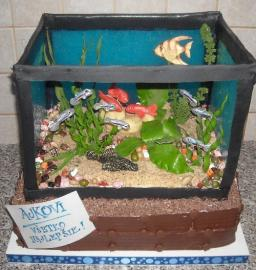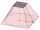# Aquarium

Aquarium is cube with edge 45 cm. How much water can fit in there?

Correct result:

V =  91.125 l

#### Solution:We would be pleased if you find an error in the word problem, spelling mistakes, or inaccuracies and send it to us. Thank you!Tips to related online calculators
Do you know the volume and unit volume, and want to convert volume units?

## Next similar math problems:

• Aquarium heightHow high does the water in the aquarium reach, if there are 36 liters of water in it? The length of the aquarium is 60 cm and the width is 4 dm.
• Cube and waterHow many liters of water can fit into a cube with an edge length of 0.11 m?
• Cubes into cuboidHow many 12 centimeter cubes fit into the block (cuboid) with 6dm, 8,4dm and 4,8?
• AquariumCan 30 liters of water fit in a cuboid aquarium with dimensions a = 3dm b = 6dm c = 5dm?
• Aquarium 6How high is the water level in the aquarium with a rectangular base 40cm and 50cm if it is filled 0,65hl of water?
• AquariumHow many liters of water can fit in an aquarium measuring 30, 15 and 20 cm?
• AquariumAquarium is rectangular box with square base containing 76 liters of water. Length of base edge is 42 cm. To what height the water level goes?
• CubesMost how many cubes with an edge length of 5 cm may fit in the cube with the inner edge of 0.4 m?
• Water reservoirThe water tank has a cuboid with edges a= 1 m, b=2 m , c = 1 m. Calculate how many centimeters of water level falls, if we fill fifteen 12 liters cans.
• Runcated pyramid teapotThe 35 cm high teapot has the shape of a truncated pyramid with the length of the edge of the lower square base a=50 cm and with the edges of the rectangular base b: 20 cm and c: 30 cm. How many liters of water will fit in the teapot?
• Children poolThe bottom of the children's pool is a regular hexagon with a = 60 cm side. The distance of opposing sides is 104 cm, the height of the pool is 45 cm. A) How many liters of water can fit into the pool? B) The pool is made of a double layer of plastic film
• Swimming poolThe swimming pool has the shape of a block with dimensions of 70dm, 25m, 200cm. How many hl of water can fit into the pool?
• PoolMr. Peter build a pool shape of a four-sided prism with rhombus base in the garden. Base edge length is 8 m, distance of the opposite walls of the pool is 7 m. Estimated depth is 144 cm. How many hectoliters of water consume Mr. Peter to fill the pool?
• MilimetersThe pool is 6 meters long, 3 meters wide and the water in it is filled with water to a height 1.7 m. When John jumped into it and completely submerged, the level has risen by 5.4 mm. How much weight John when we know that one liter of the human body weigh
• How muchHow much money will we pay for 20 planks 4m long, 15cm wide and 26mm thick when 1m³ of wood costs 4500kč?
• Volume per timeHow long does fill take for a pump with a volume flow of 200 l per minute fill a cube-shaped tank up to 75% of its height if the length of the cube edge is 4 m?
• HectolitersHow many hectoliters of water fits into cuboid tank with dimensions of a = 3.5 m b = 2.5 m c = 1.4 m?